# And life? Life will go on.

Originally I planned on showing you some numerical results from the circular 4D numbers while explaining there is also a number alpha in 4D. For me that would be a nice holiday away from all that 4D complex number stuff from the last months…

But the numerical applet did not work, it is still dead in the water:

Ok ok, I could have done those numerical showings also in rigid analysis but I guessed that calculating a 4D tau for circular numbers via analysis was too much. And I settled for a much more easy to understand thing:

The logarithmic function for every 2D circular number. In the field of professional math professors the 2D circular numbers are known as the split complex numbers.

So that is what the next post will be about: Finding log(z) for all invertible split complex numbers.

I only wrote one previous post on the 2D circular aka split complex numbers and that dates back to Nov 24 of the year 2016:

The second hybrid: a 4D mix of the complex and the circular plane.

Ha ha, now I can laugh about it but back in the time it was some hefty pain. Anyway to make a long story short: In that old post from 2016 I calculated the log for just one split complex number namely the first imaginary unit j.

Let me show you my favorite part of that old post from 2016: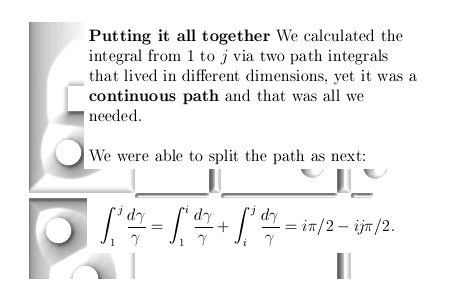So the next update will only contain 2×2 size matrices while I skip the detail that the log lives mainly in the hybrid number system from the old post.

# Part 20: On the structure of non-invertible 4D complex numbers.

In general it is rather hard to find non-invertible 4D complex numbers because the determinant is non-negative everywhere. Just try it yourself, write down just one 4D complex number that is non-zero and not invertible.

That is not an easy task, after some time you will find some but do you have all?

But if you understand the concept of the eigenvalues that every 4D complex number Z has, it is easy to understand that if a 4D complex number is non-invertible at least one of the eigenvalues must be zero.

In previous posts we already unearthed the four eigenvalue functions that return the four eigenvalues each 4D complex number has.

In this post we will try to find where these eigenvalue functions are zero.

Since eigenvalue functions come in pairs whenever possible in the case of 4D complex numbers we only have two pairs of those eigenvalue functions.

Let’s stop the talking and just post the twelve pictures that make up part number 20 into the basics of the four dimensional complex numbers.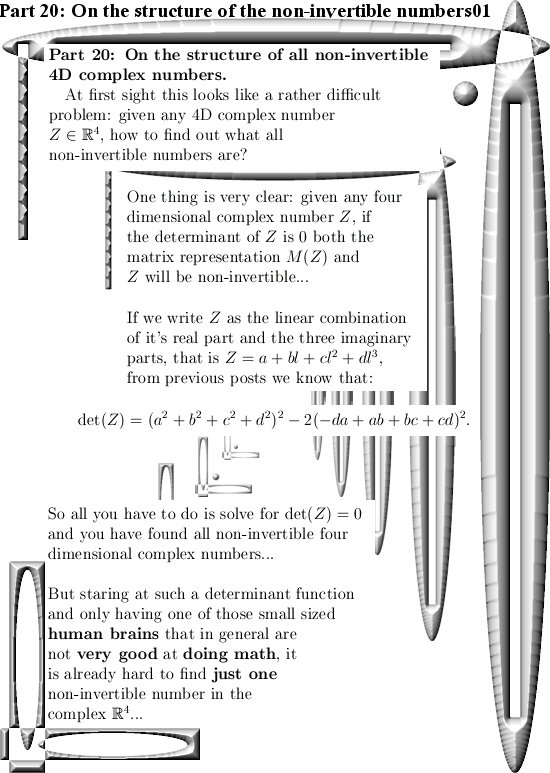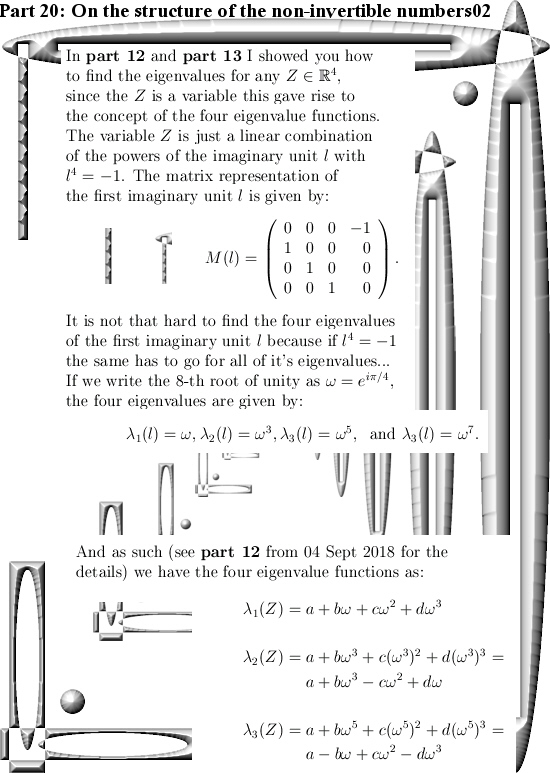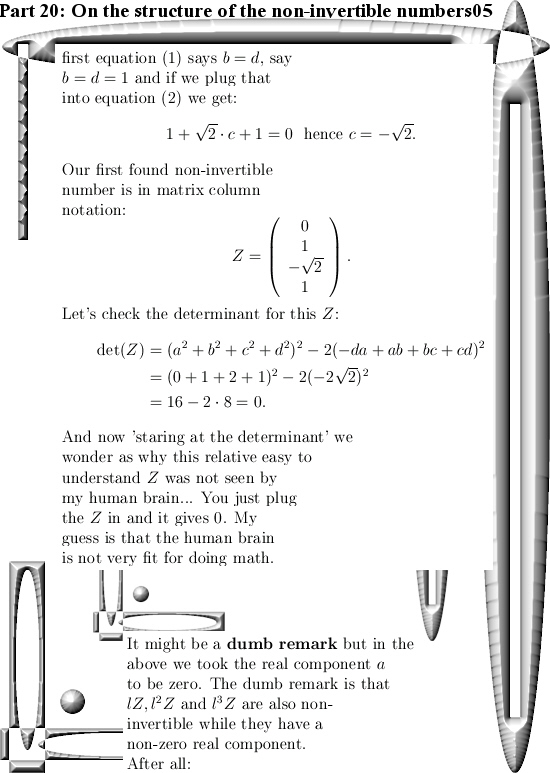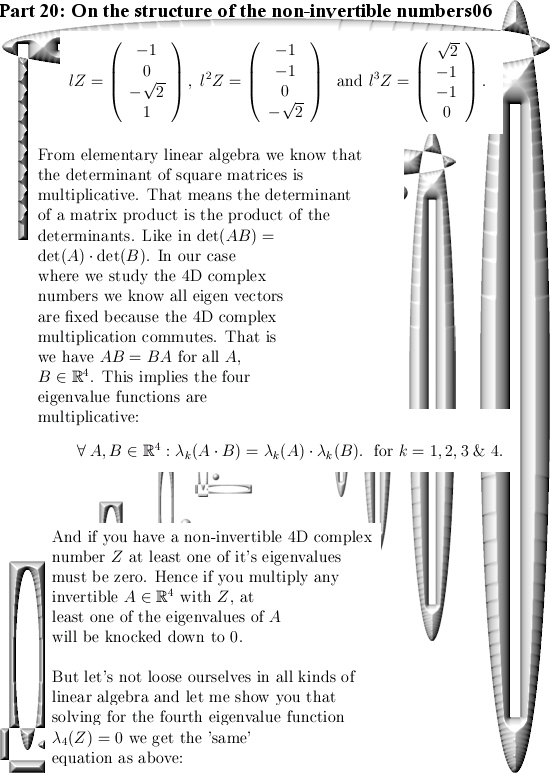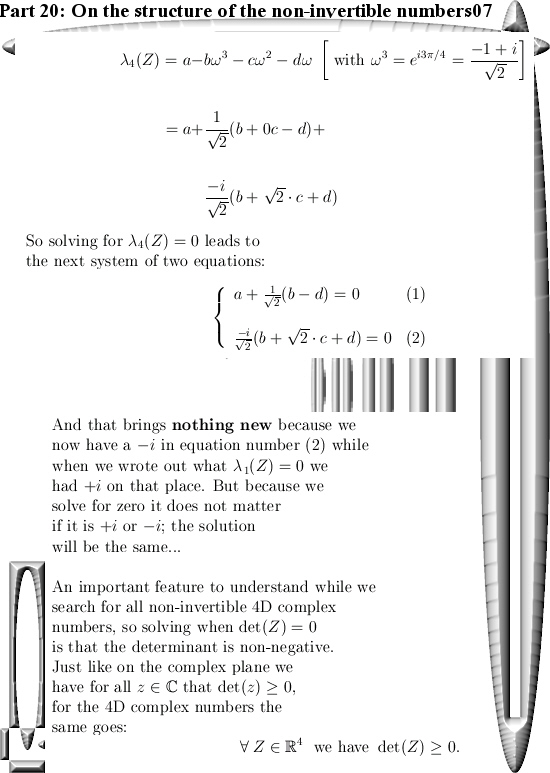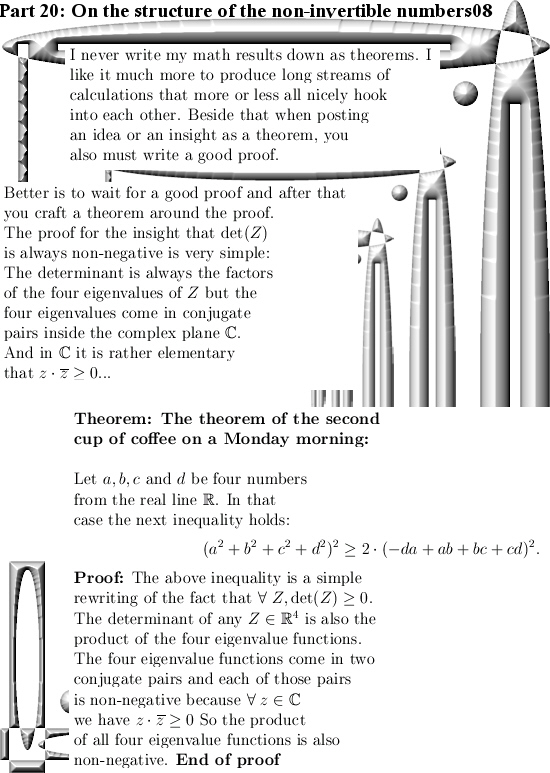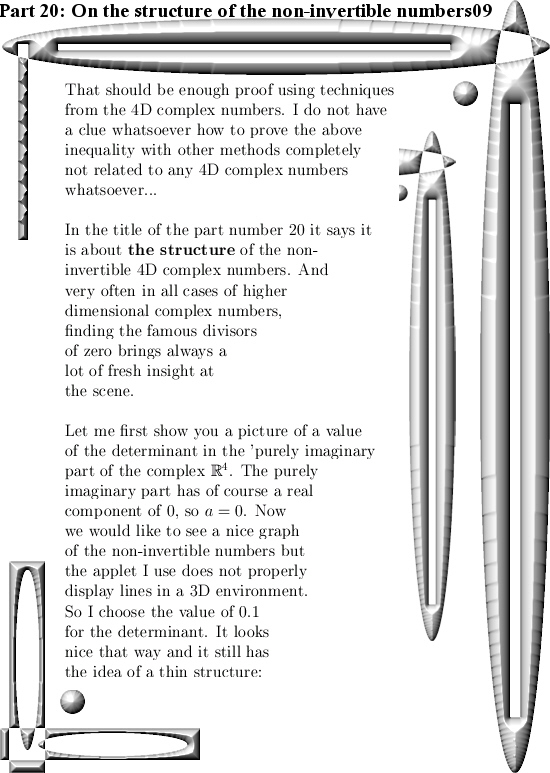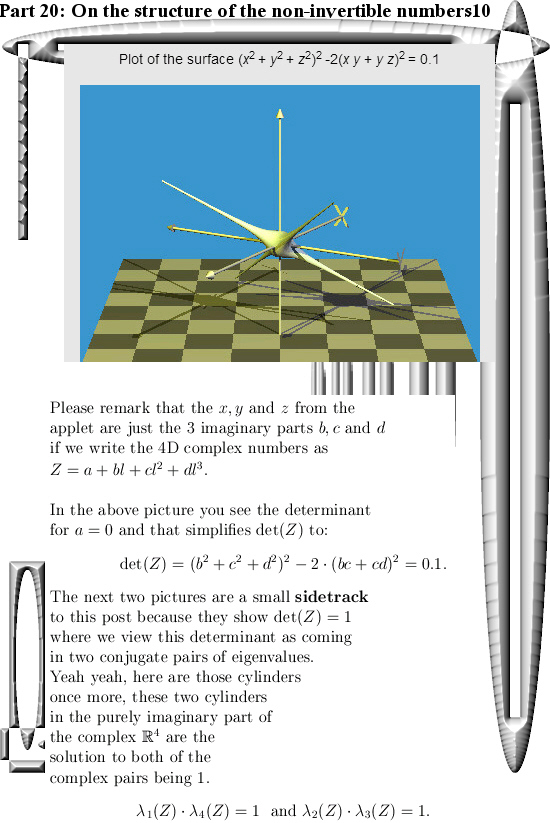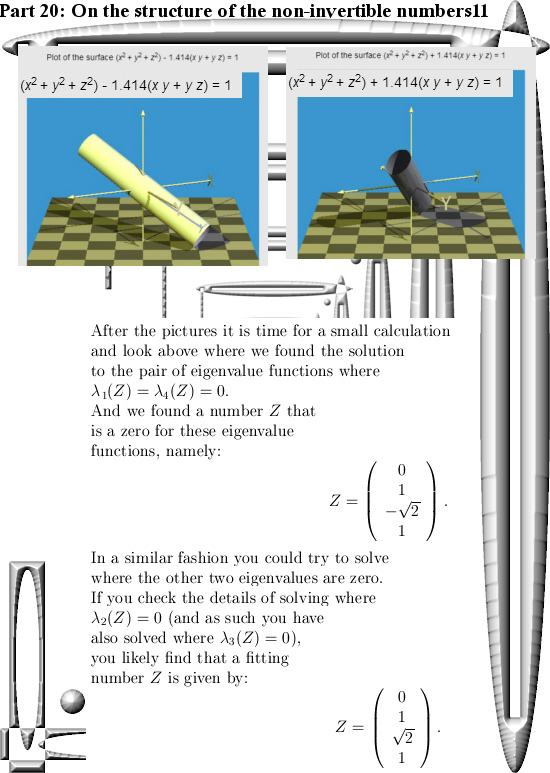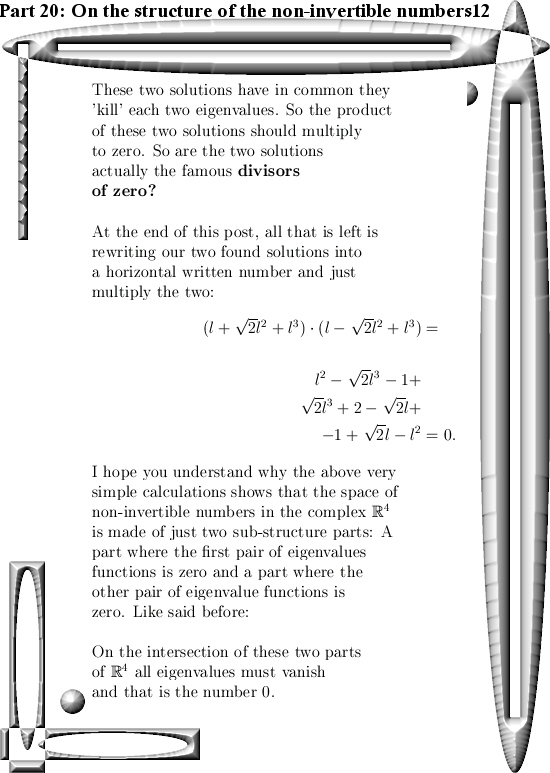Ok, that was it for part 20 in the series that covers the basics of 4D complex numbers.

See you around my dear reader.